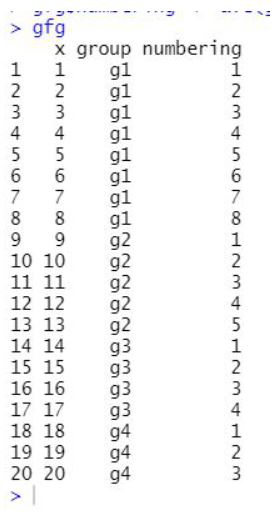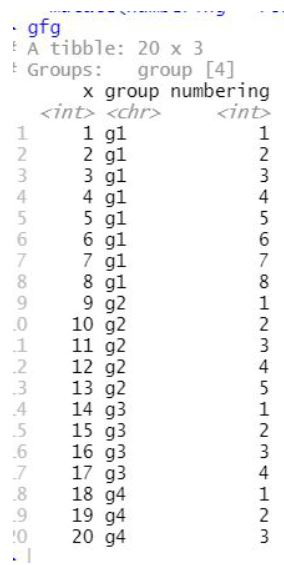# Numbering Rows within Groups of DataFrame in R

• Last Updated : 23 Sep, 2021

In this article, we will discuss how to number rows within the group of the dataframe in the R programming language

## Method 1: Using ave() function

Call the ave() function, which is a base function of the R language, and pass the required parameters to this function and this process will be leading to the numbering rows within the group of the given dataframe in the R programming language.

ave() function is used for subsets of x[] are averaged, where each subset consist of those observations with the same factor levels.

Syntax:

ave(x, …, FUN = mean)

Parameters:

• x: A numeric.
• … : Grouping variables, typically factors, all of the same length as x.
• FUN: Function to apply for each factor level combination

Example: Numbering rows within groups

## R

 `gfg<-``data.frame``(x=1:20,group=``c``(``rep``(``"g1"``, 8),``                               ``rep``(``"g2"``, 5),``                               ``rep``(``"g3"``,4),``                               ``rep``(``"g4"``,3)))`` ` `gfg\$numbering <- ``ave``(gfg\$x,gfg\$group,FUN = seq_along)`` ` `gfg`

Output:## Method 2: Using mutate() function from dplyr package

In this approach for numbering rows within the group of the dataframe using ave function, the user needs to install and import the dplyr package in the working R console, here this package is required to import because the function mutate() is the function present in this particular library, then the user needs to call the mutate() function with the required parameter passed into it, to get the numbering rows within the group of the given dataframe in the R programming language.

mutate() function is used to mutate adds new variables and preserves existing; transmute drops existing variables.

Syntax:

mutate(.data, …)

Parameters:

• .data: A tbl. All main verbs are S3 generics and provide methods for tbl_df, tbl_dt and tbl_sql.
• … : Name-value pairs of expressions. Use NULL to drop a variable.

Example: Numbering rows within groups

## R

 `library``(``"dplyr"``)`` ` `gfg<-``data.frame``(x=1:20,group=``c``(``rep``(``"g1"``, 8),``rep``(``"g2"``, 5),``                               ``rep``(``"g3"``,4),``rep``(``"g4"``,3)))`` ` `gfg <- gfg %>%                            `` ``group_by``(group) %>%`` ``mutate``(numbering = ``row_number``())`` ` `gfg`

Output:My Personal Notes arrow_drop_up